# PROMOTER TOOL

Since we wanted to design our Listeria detection system to work both E. coli (for testing) and in Lactococcus lactis (for the industrial application), we wanted to select a promoter that would work well in both species. We came across a paper by Jensen and Hammer, who designed a series of 37 constitutive promoters and characterised their activity in both E. coli and L. lactis using a beta galactosidase assay. They provided an image of aligned sequences juxtaposed with their activity in both species, and this made us wonder whether we could selectively alter certain parts of these sequences to optimise their activity for our purposes. We realised this would mean designing a more active constitutive promoter for our agrC and agrA sensing components, as we want these to be expressed all the time and in large amounts. However, this tool could be used by other iGEM teams who may want a promoter associated with a lower constitutive expression to keep readthrough expression levels at a low level (for more information, see our Collaborations Page)

#### How does our model work?

1. Each sequence was hand-typed into an Excel file and, for ease of comparison, the sequences were aligned and if there was a deletion, it was indicated with “-“.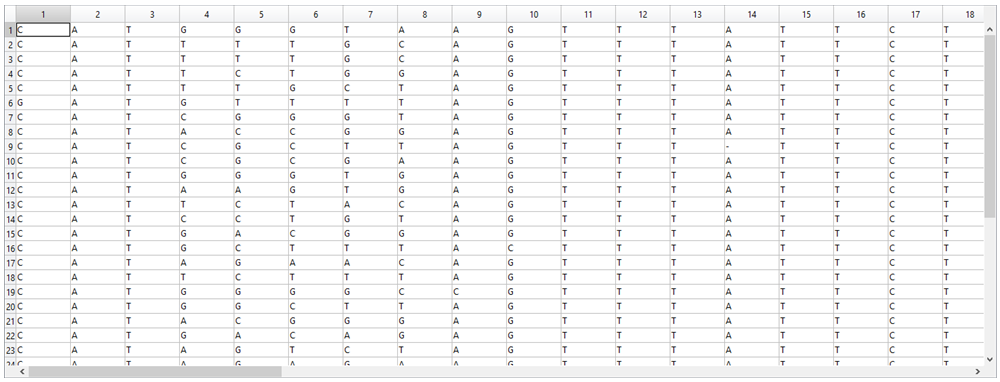2. Once the program is started in Matlab, the first pop up window you see is this: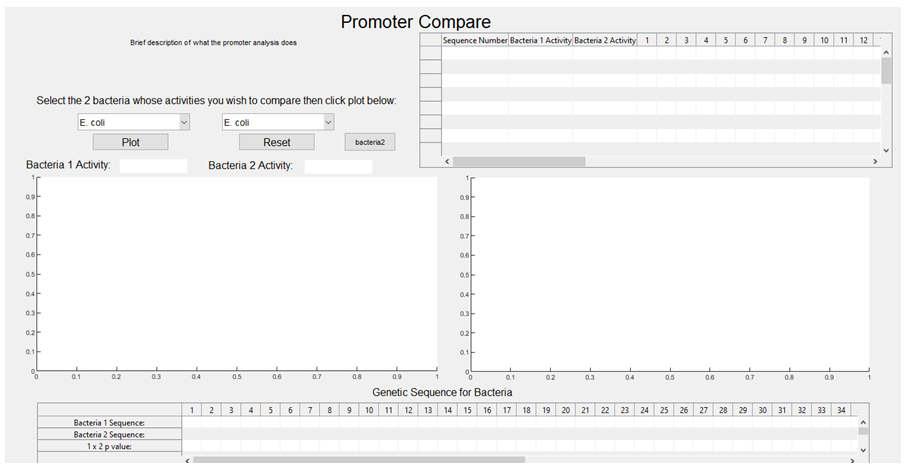3. The activities associated with each sequence are plotted against each other, with E. coli on the x-axis and L. lactis on the y-axis (the graph is then stored and will pop up upon the user selecting E. coli and L. lactis from the graphical user interface (GUI) dropdown boxes, with a regression line also plotted).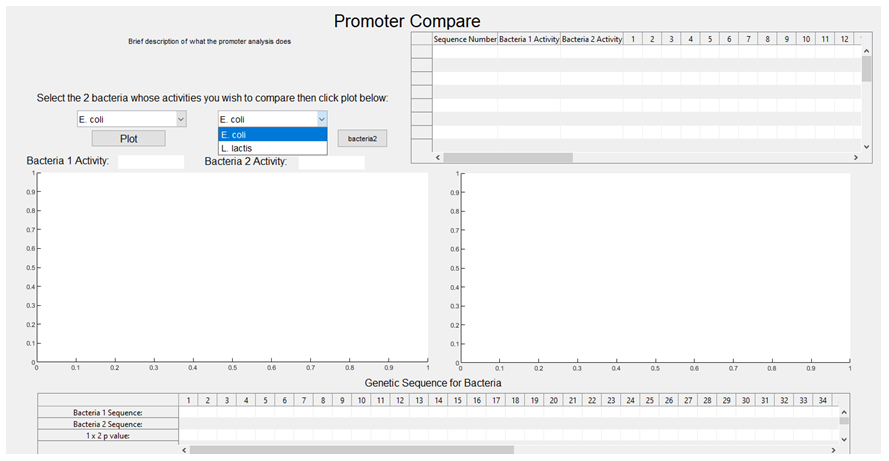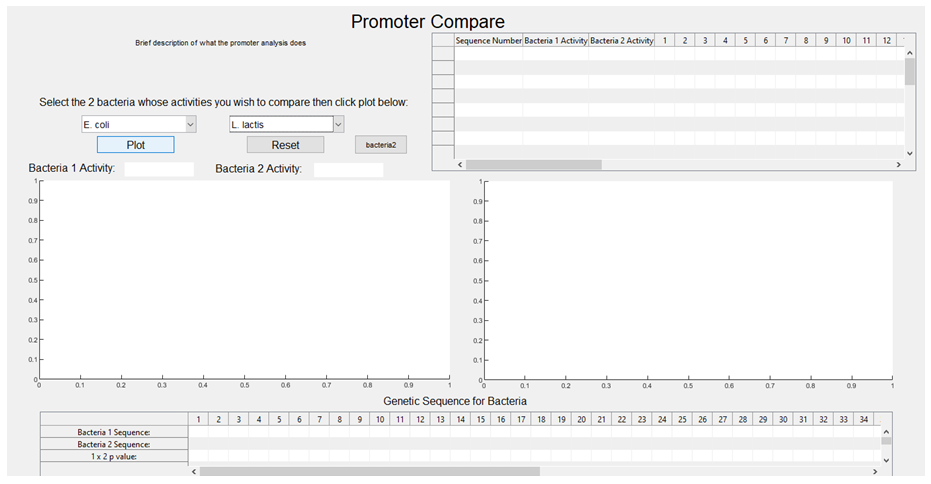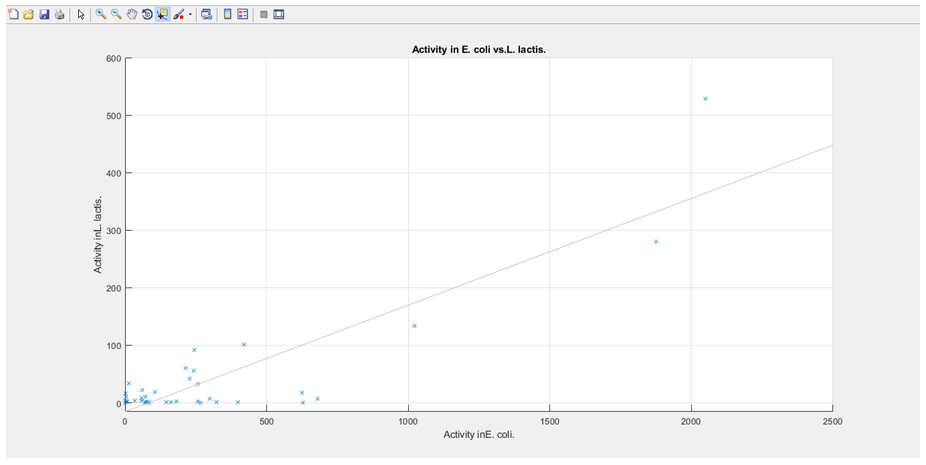4. We determined the frequency of each nucleotide in a particular column (e.g.: in column 1 94.59% of nucleotides were a “C”, 2.7% were “G”, 2.7% were a “T”, and there were no “A” or “-“).

5. The end result is a large table comparing the frequencies of each nucleotide in a particular position (column) across the 37 sequences, which we called CPactivity.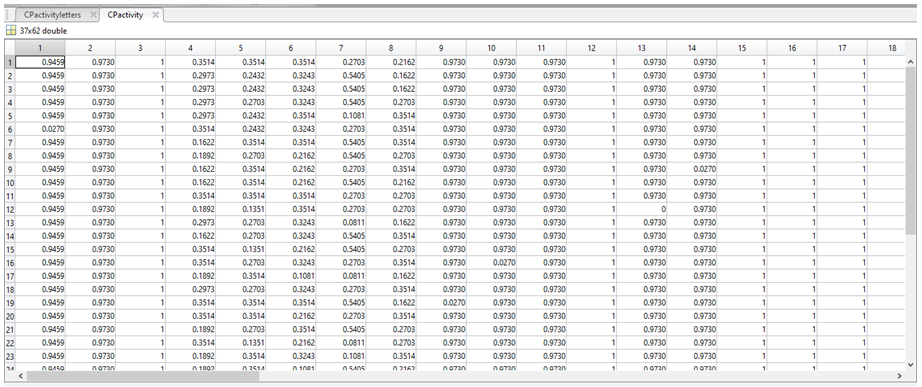6. We then used the following equation: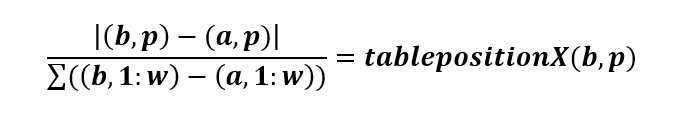(b, p) = coordinate 1, where b can be any value row index between 1 and 37 (i.e. the total number of sequences) and p is any column index between 1 and 60
(a, p) = coordinate 2, where a can be any value row index between 1 and 37 (i.e. the total number of sequences) and does not have to be equal to b; however, if b=a, a NaN value will be generated.
(b, 1:w) = the sum of the values in row b
(a, 1:w) = the sum of the values in row a
Note: coordinates are written as (row, column)

In MatLab, we used this equation to compare sequence 1 to itself and every other sequence, then sequence 2 to itself and every other sequence and so on. This calculation resulted in the less common bases being awarded a higher weight, as we theorised that if the activity is changing across all sequences, yet an area is conserved (i.e. all T’s) then this region is less likely to be responsible for any changes between the overall sequence activity. The resulting values were then stored in a set of new variables (each a 37 by 37 table). The table for column 1 is called tablepositionX, which is shown below.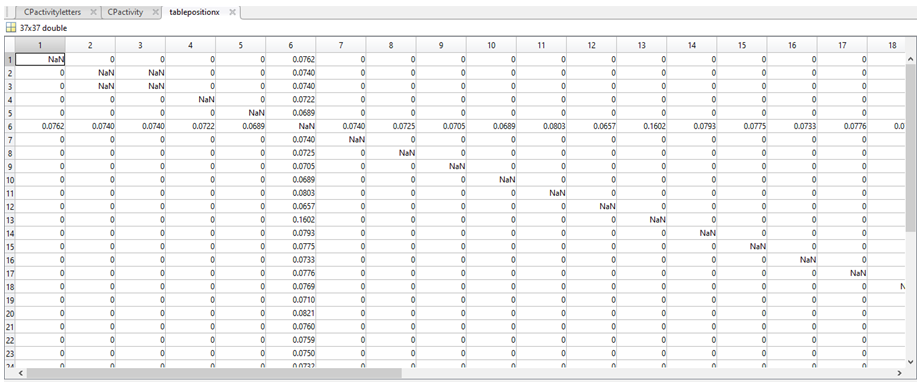7. The non zero value means from tablepositionX were then collated into a new variable called averageactivitychange, to assign a mean “weight” associated with each nucleotide.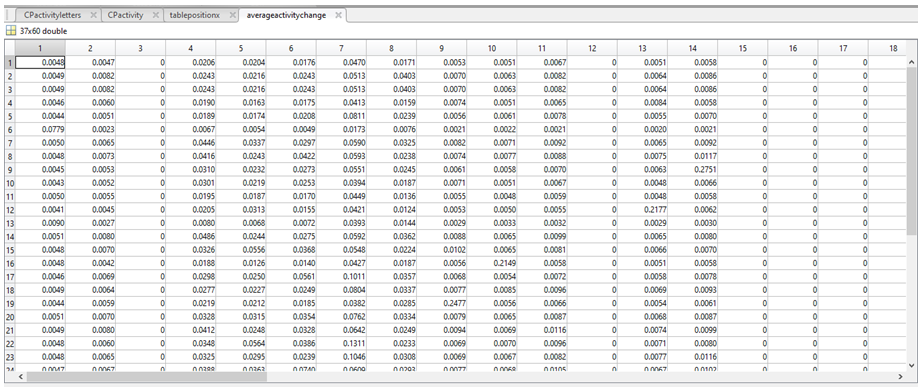8. Each base is now associated with a comparative frequency (as a fraction), with the less common bases having a higher weighting, (the sum of each row is 1 at this point in time). We then used the following equations: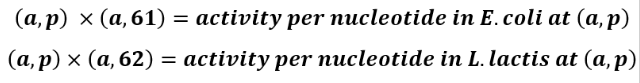a can be any row indices between 1 and 37
p can be any column indices between 1 and 60.
column 61 contains the activities of each promoter sequence in E. coli
column 62 contains the activities of each promoter sequence in L. lactis

9. We stored the variable “activity per nucleotide in E. coli” as a 37 by 60 table called activitychangebacteria1, and the variable “activity per nucleotide in L. lactis” also as a 37 by 60 table called activitychangebacteria2.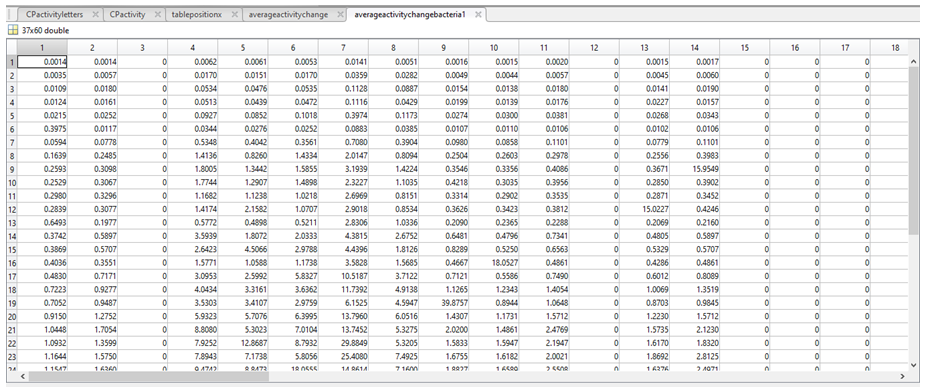10. We then aimed to acquire a value per nucleotide in a given column (p) of activitychangebacteria1 and activitychangebacteria2 (to tell us the relative activity associated with having a A in position 1 of the promoter, for example). To do this we first had to verify the presence of a particular nucleotide (N*) in column p, followed by the total number of a given nucleotide in column p (length(N)). If the length(N) was greater than 1 (i.e there is more than 1 of a given nucleotide in column p), then a mean activity of every “N” in activitychangebacteria1 (for column p) and activitychangebacteria2 (for column p) was calculated and stored in either averageactivityperbasebacteria1 or averageactivityperbasebacteria2. If length(N) was less than 1, a value of 0 was assigned to every N in column p and stored in either averageactivityperbasebacteria1 and averageactivityperbasebacteria2.

*NOTE: “N” refers to “A”, “C”, “G”, “T”, or “-” p can be any column index between 1 and 60

11. Each row in averageactivityperbasebacteria1 (first image below) and averageactivityperbasebacteria2 (second image below) corresponds to a nucleotide (1 = A, 2 = C, 3 = G, 4 = T, 5 = “-“).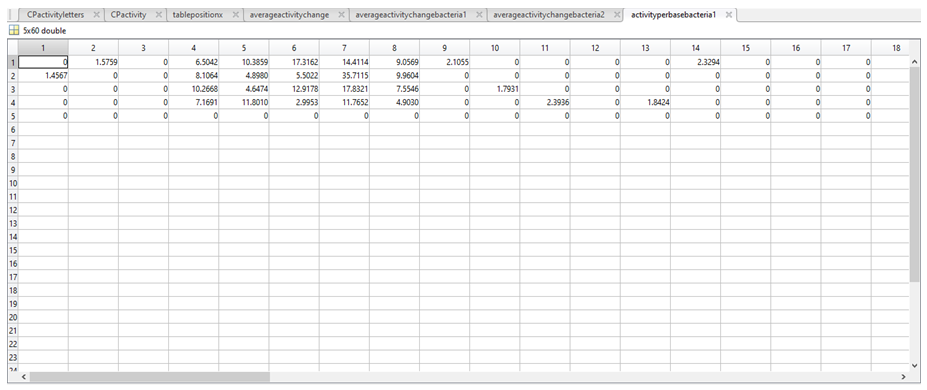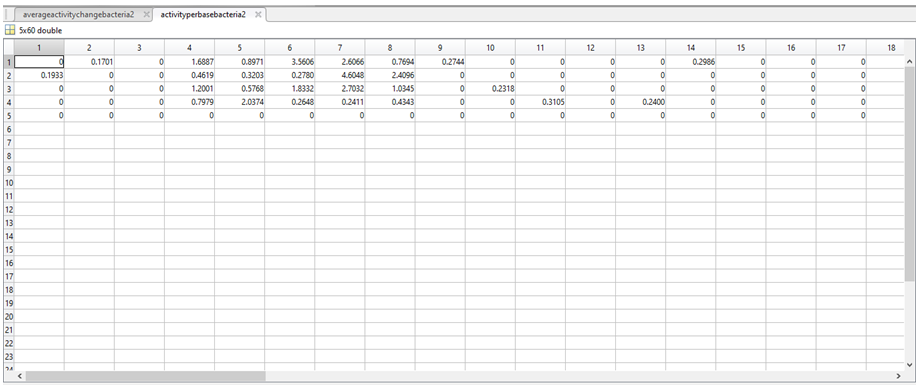12. To determine if there was any significant difference between the activities assigned in the two above variables, a t-test was carried out in MatLab to produce a number between 0 and 1 representing the statistical significance of the difference between the above two variables. The results of the t-test were stored in a further two variables called siglev1 and siglev2. Each row in siglev1 and siglev2 also corresponds to a nucleotide (1 = A, 2 = C, 3 = G, 4 = T, 5 = “-“).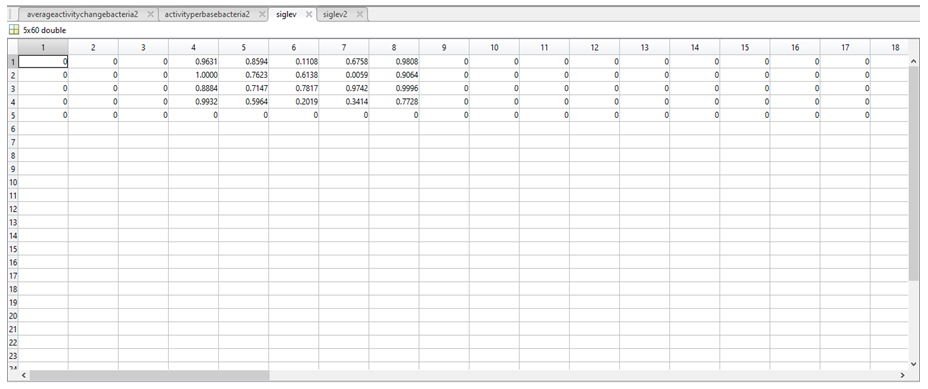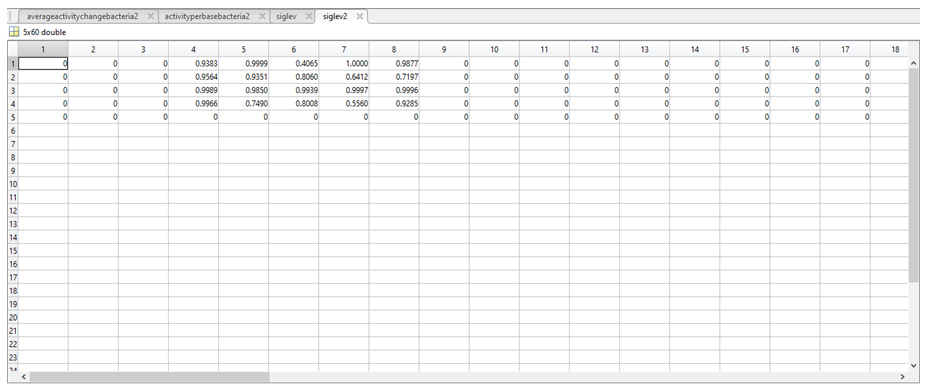13. The siglev variables are then visualised on the GUI using the imagesc function, (insert image of GUI plots), with white areas indicating a lack of data to carry out the t test at this particular position, and a gradient from blue to orange, with blue representing the areas of highest significance and areas of orange representing areas of lower significance.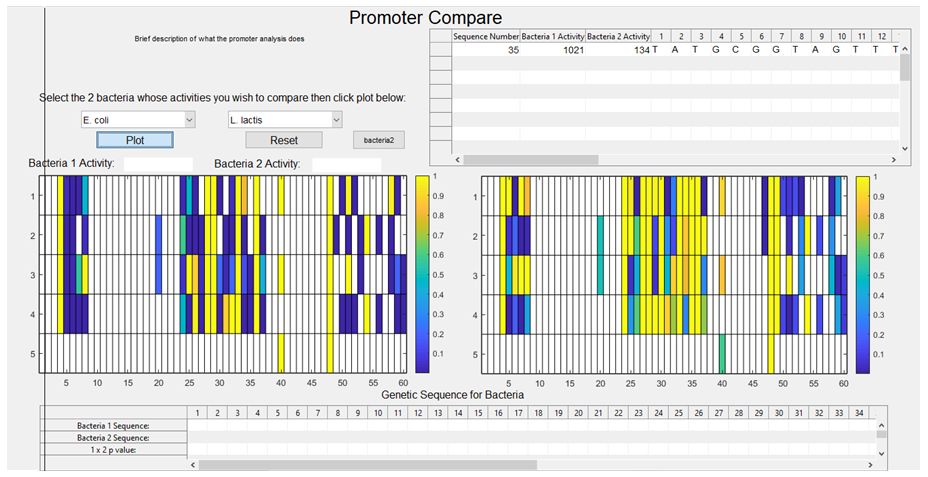### Discussion

The purpose behind designing this tool in the GUI app in MatLab was so that a user could, with ease, design a new synthetic promoter using pre-existing data on characterised promoters within a particular (pair of) species. To that end, we wanted our program to be able to display the activity associated with a particular base in a particular column upon the user left-clicking a particular cell from the imagesc plot. This would allow the user to determine the effect on the overall activity of the sequence if a substitution took place at a region of high significance. We then wanted a user to be able to design their new sequence within our program upon a right-click, storing the nucleotide associated with the particular cell the user selected.

The purpose of having bacterial selection drop down options on the GUI was so that, in the future, the sequences may have their activities measured across other species and this data could be integrated into our model. We also added a function to allow the user to select a sequence of interest (say, one that has high activity in both species being compared) and store this sequence for reference when designing the new sequence on the GUI. Additionally, we created the variables W and L which correspond, respectively, to the number of columns and rows in the initial Excel file, so that, in the future, additional sequences of variable lengths (not just 60nt) could be integrated into our model.